# Chebyshev Polynomials: Definition, History & Properties

Instructor: Gerald Lemay

Gerald has taught engineering, math and science and has a doctorate in electrical engineering.

This lesson looks at the remarkable Chebyshev polynomials and defines them using properties of recursion and orthogonality. Chebyshev polynomials have applications in science and engineering.

## The Polynomials of Pafnuty Chebyshev

An extraordinary 19th Russian mathematician named Pafnuty Chebyshev had an uncommon way of thinking about the relationship between math theory and science applications. As students of math, we often ask ''What is this good for?'' or ''How can this math be used?'' Rather than viewing applications as the beneficiary of elegant mathematics, Chebyshev had a broader idea. He would see a wonderful application and ask if the math adequately described the science. In fact, he found many of his greatest theoretical mathematical discoveries by observing mechanical systems (like steam engines). When the mathematical theory at the time fell short of adequately explaining how an application worked, this inspired Chebyshev to create better math theory. It is out of this mindset he extended the idea of orthogonal polynomials to a set of polynomials that now bear his name. Fast forward to our present time and we find the Chebyshev polynomial intimately linked with digital filters, matching networks and other modern communication systems. There may be a Chebyshev filter in your smart phone or tablet!

## Orthogonal Polynomials

Deciphering a word helps with understanding. What about the word polynomial? If you accept the word nomial as being a single term like 1, x or x2 then a polynomial is ''many'' of these terms together like 1 + x. We will get back to the word ''orthogonal'' later in this lesson. For now lets look at the Chebyshev polynomials.

The degree of a polynomial is the highest exponent of the variable in any of the terms.

The zeroth degree Chebyshev polynomial, To is: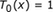When x is raised to the 0 power, the degree is 0. And x0 = 1.

By the way, Chebyshev's name is translated with a T instead of a C in some languages. That's why the letter ''T'' is used for his polynomials.

It is easy to plot To: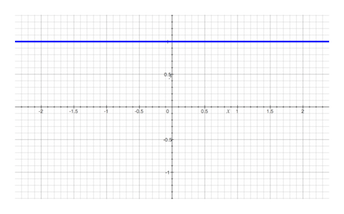This is the zeroth-degree Chebyshev polynomial. How about a first-degree polynomial? Right, the highest power of x in any of the terms will be 1. The next example is Chebyshev's first-degree polynomial: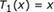Let's plot T1 and To on the same graph.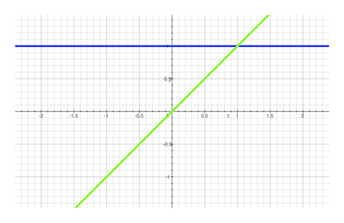A nice property of the Chebyshev polynomials is we can generate the rest of the Chebyshev polynomials using just these first two. The equation that tells us how to do this, is called a recursion equation. It's a way to get the next Chebyshev polynomial if we know the two previous ones. The Chebyshev recursion equation is, in words:

• Multiply the current polynomial by 2x
• From this result, subtract the previous polynomial

For example, to get T2

• Multiply T1 (which is x) by 2x giving 2x2
• From 2x2 subtract To

Thus,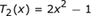Please take a moment and try calculating T3 on your own.

How did you do? To find T3, take 2x times T2 and then subtract T1.

This gives us 2x(2x2 - 1) - x which simplifies to: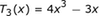Plotting these first four Chebyshev polynomials.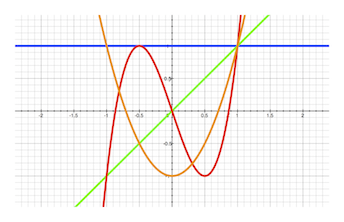Instead of generating and plotting more and more of the Chebyshev polynomials, we will use what we have so far to delve into some fascinating properties. But first, let's look at the general way to write the recursion: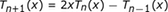Tn+1 is the next Chebyshev polynomial to find. The recursion steps are to multiply the current one, Tn, by 2x and subtract the previous one, Tn - 1.

## The Orthogonal Property of the Chebyshev Polynomials

As promised, lets delve into the word orthogonal. The word ''ortho'' means straight or right. When taken together with ''gonal'' we are describing two perpendicular lines that form a right angle.

A right angle is an angle of 90o. The cosine of 90o is zero. This is our test for orthogonality. Measure the angle between two lines, compute the cosine of this angle, and if we get 0, then the lines are orthogonal.

To unlock this lesson you must be a Study.com Member.

### Register to view this lesson

Are you a student or a teacher?

### Unlock Your Education

#### See for yourself why 30 million people use Study.com

##### Become a Study.com member and start learning now.
Back
What teachers are saying about Study.com

### Earning College Credit

Did you know… We have over 160 college courses that prepare you to earn credit by exam that is accepted by over 1,500 colleges and universities. You can test out of the first two years of college and save thousands off your degree. Anyone can earn credit-by-exam regardless of age or education level.